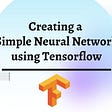# Machine learning

Model = Algorithms(Data)

There is also error (e) that is independent of the input data (X).

Y = f(X) + e

This error might be error such as not having enough attributes to sufficiently characterize the best mapping from X to Y . This error is called irreducible error because no matter how good we get at estimating the target function (f), we cannot reduce this error. This is to say, that the problem of learning a function from data is a difficult problem and this is the reason why the field of machine learning and machine learning algorithms exist. Much time in applied machine learning is spent attempting to improve the estimate of the underlying function and in term improve the performance of the predictions made by the model.

Different machine learning algorithms make different assumptions about the shape and structure of the function and how best to optimize a representation to approximate it. This is why it is so important to try a suite of different algorithms on a machine learning problem, because we cannot know before hand which approach will be best at estimating the structure of the underlying function we are trying to approximate.

# Parametric and nonparametric machine learning algorithms

• Parametric methods make large assumptions about the mapping of the input variables to the output variable and in turn are faster to train, require less data but may not be as powerful.
• Nonparametric methods make few or no assumptions about the target function and in turn require a lot more data, are slower to train and have a higher model complexity but can result in more powerful models.

# Supervised, unsupervised and semi-supervised learning

• Supervised: All data is labeled and the algorithms learn to predict the output from the input data. Classification: A classification problem is when the output variable is a category, such as red or blue or disease and no disease. Regression: A regression problem is when the output variable is a real value, such as dollars or weight.
• Unsupervised: All data is unlabeled and the algorithms learn to inherent structure from the input data. These are called unsupervised learning because unlike supervised learning above there is no correct answers and there is no teacher. Algorithms are left to their own devises to discover and present the interesting structure in the data. Clustering: A clustering problem is where you want to discover the inherent groupings in the data, such as grouping customers by purchasing behavior. Association: An association rule learning problem is where you want to discover rules that describe large portions of your data, such as people that buy A also tend to buy B.
• Semi-supervised: Some data is labeled but most of it is unlabeled and a mixture of supervised and unsupervised techniques can be used. A good example is a photo archive where only some of the images are labeled, (e.g. dog, cat, person) and the majority are unlabeled. Many real world machine learning problems fall into this area. This is because it can be expensive or time consuming to label data as it may require access to domain experts. Whereas unlabeled data is cheap and easy to collect and store. You can use unsupervised learning techniques to discover and learn the structure in the input variables. You can also use supervised learning techniques to make best guess predictions for the unlabeled data, feed that data back into the supervised learning algorithm as training data and use the model to make predictions on new unseen data.

# Bias-variance trade-off for machine learning algorithms

• Bias is the simplifying assumptions made by the model to make the target function easier to approximate.
• Variance is the amount that the estimate of the target function will change given different training data.
• Trade-off is tension between the error introduced by the bias and the variance.
• Irreducible Error the irreducible error cannot be reduced regardless of what algorithm is used. It is the error introduced from the chosen framing of the problem and may be caused by factors like unknown variables that influence the mapping of the input variables to the output variable.

# Generalization in machine learning of overfitting and underfitting

Both overfitting and underfitting can lead to poor model performance. But by far the most common problem in applied machine learning is overfitting. Overfitting is such a problem because the evaluation of machine learning algorithms on training data is different from the evaluation we actually care the most about, namely how well the algorithm performs on unseen data. There are two important techniques that you can use when evaluating machine learning algorithms to limit overfitting:

1. Use a resampling technique to estimate model accuracy.
2. Hold back a validation dataset.

The most popular resampling technique is k-fold cross validation. It allows you to train and test your model k-times on different subsets of training data and build up an estimate of the performance of a machine learning model on unseen data. A validation dataset is simply a subset of your training data that you hold back from your machine learning algorithms until the very end of your project. After you have selected and tuned your machine learning algorithms on your training dataset you can evaluate the learned models on the validation dataset to get a final objective idea of how the models might perform on unseen data. Using cross validation is a gold standard in applied machine learning for estimating model accuracy on unseen data. If you have the data, using a validation dataset is also an excellent practice.

• Overfitting: Overfitting happens when a model learns the detail and noise in the training data to the extent that it negatively impacts the performance on the model on new data. Good performance on the training data, poor generalization to other data. 
• Underfitting: Underfitting refers to a model that can neither model the training data nor generalize to new data. The remedy is to move on and try alternate machine learning algorithms. Poor performance on the training data and poor generalization to other data.

# Why are GPU’s well suited for deep learning?

The real reason for this is memory bandwidth and not necessarily parallelism.

## More from Natarajan Santhosh

Love podcasts or audiobooks? Learn on the go with our new app.

## Reading: StairNet — Top-Down Semantic Aggregation (Object Detection)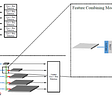## What Are Artificial Neural Networks, Really?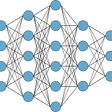## Mechanism of Action (MoA)- The Kaggle Competition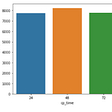## Building a Search by Image Shopping Experience with VOVA and Milvus## Two uses of TDNNs (from Wikipedia)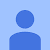## How to write a ReLU function and its derivative in python?## Creating a simple neural network using TensorFlow